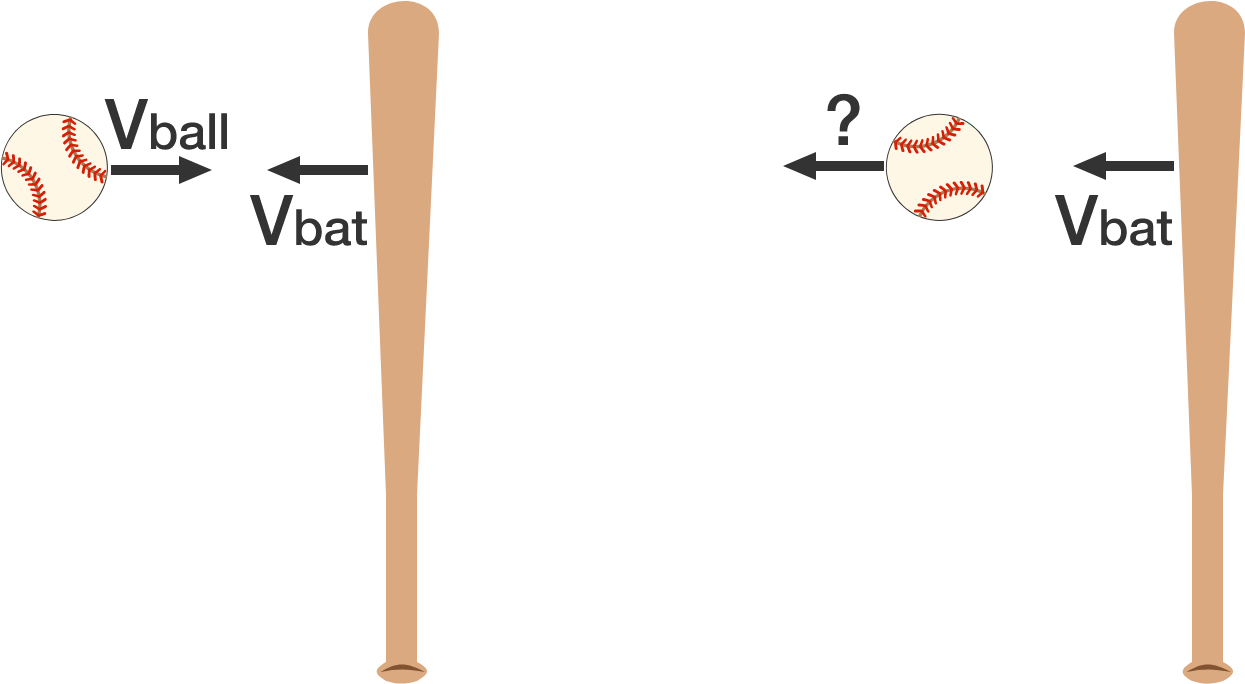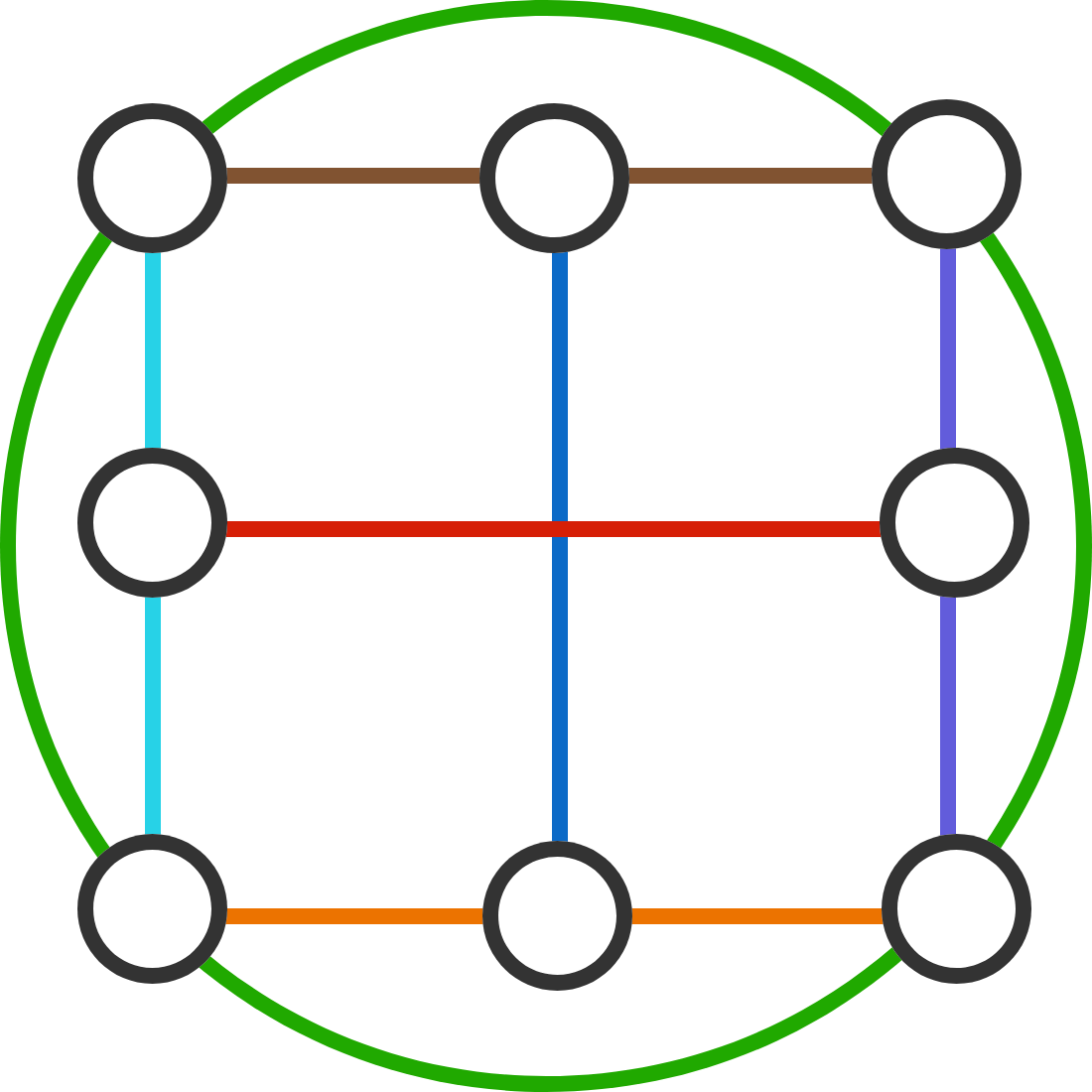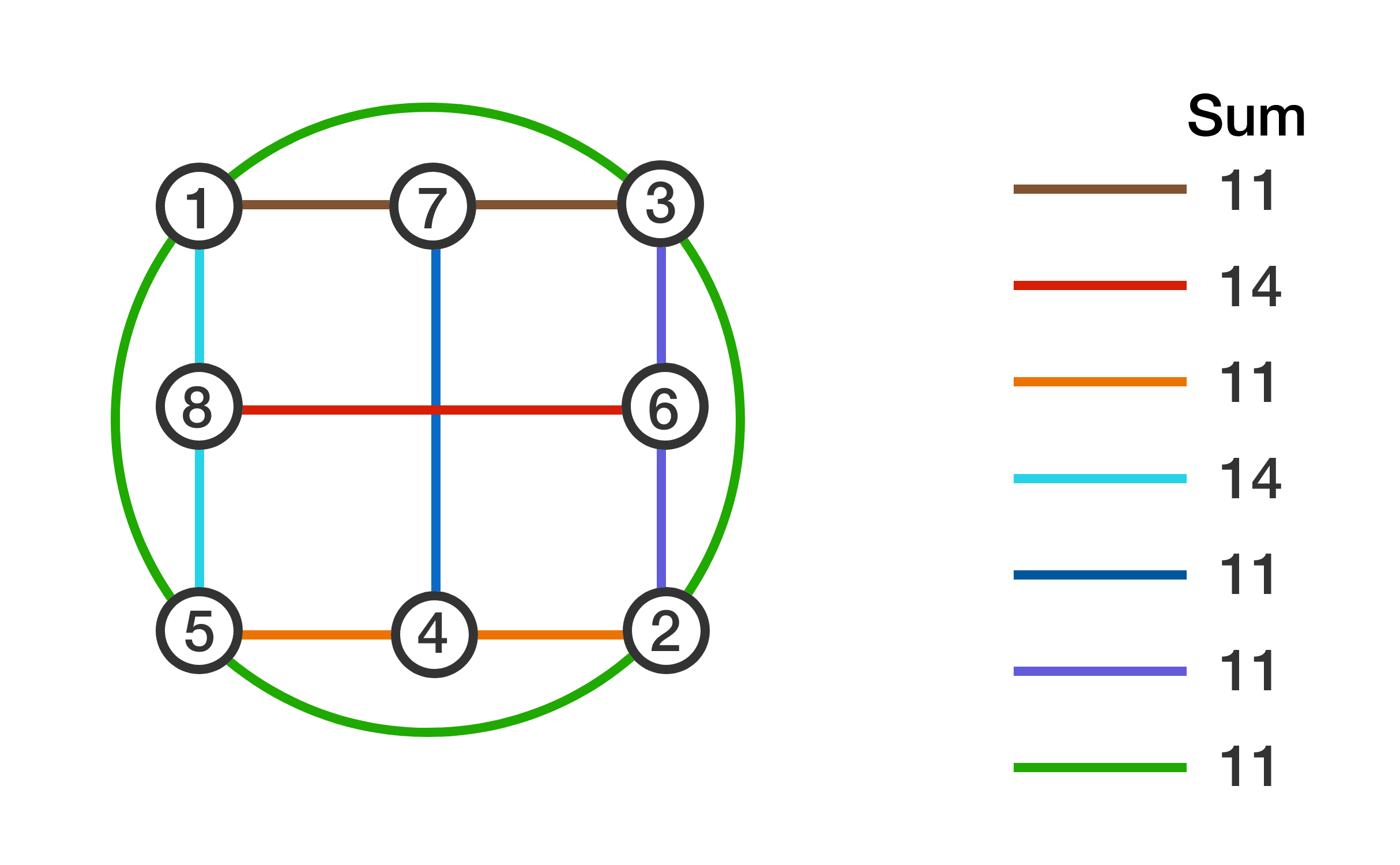# Problems of the Week

Contribute a problem

# 2017-07-17 Basic

You find a forgotten list on an ancient piece of paper while cleaning your professor's office. It has the following written down:

Exactly 1 statement on this list is false.
Exactly 2 statements on this list are false.
Exactly 3 statements on this list are false.
Exactly 4 statements on this list are false.

How many statements on the list are in fact false?

Find the value of

$\frac { { \left( { 3 }^{ 2017 } \right) }^{ 2 }-{ \left( { 3 }^{ 2015 } \right) }^{ 2 } }{ { \left( { 3 }^{ 2018 } \right) }^{ 2 }-{ \left( { 3 }^{ 2016 } \right) }^{ 2 } }.$A ball approaches a batsman horizontally at speed $v_{\text{ball}}$, and the batsman swings the bat, hitting the ball back along the same path. If the speed of the bat is $v_{\text{bat}}$ when it hits the ball, then what is the recoil speed of the ball?


Details and Assumptions:

• The collision is perfectly elastic.
• For all practical purposes, the speed of the bat is constant throughout the collision.

True or False?

For any integer $n>3$, the last digit of $\large 2^{1\times 2\times 3\times \cdots \times n}$ is always 6.Can we place a distinct integer from 1 to 8 into each black circle such that the sums along the 7 colored paths are the same?

For example, we have filled up the circles, but not all of the colored paths have the same sum.Inspiration.

×